# Unified Modeling Language

The Unified Modeling Language is a standard of specifying, visualizing, documenting modeling language used for:

·         Design, analysis and implementation

·         Modeling business and other process.

The Unified Modeling language was developed by Object Management Group in 1997. UML is a language for business analyst, Software architects and it is also used by the developers for describing, specifying, documenting, etc.  UML is process independent and could be applied in the context of different processes.

##### Types of UML diagram

UML diagram is divided into two types: Structural UML diagram and behavioral UML diagram

##### Structural UML diagram

·         Class diagram

·         Object diagram

·         Package diagram

·         Component diagram

·         Composite structural diagram

·         Deployment diagram

##### Behavioral UML diagram

·         Activity diagram

·         Sequence diagram

·         Use case diagram

·         State diagram

·         Communication diagram

·         Interaction overview diagram

·         Timing diagram

##### Class diagram

Class diagram is used to represents the static view of an application. Class diagram are backbone of nearly every object oriented programming. This diagram is described as the static structure of a system. It shows relationship between attributes, object, classes and operations.

Class diagram are used for many purposes, including detailed design modeling and both conceptual/ domain modeling. The class diagrams are the only diagram in UML which can be mapped directly with object oriented languages and so they are widely used at time of constructions. Following is an example of class diagram: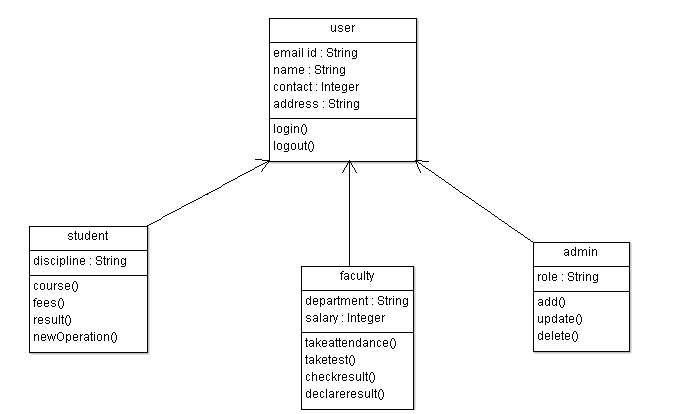##### Above is an example of college management system which is described as:

A user is a base class which has three derived class: student, faculty and admin. These three classes inherit the properties and methods of base class or parent class.

##### Object diagram

Object diagram are sometimes also known as instance diagram. As object diagram are derived from class diagram so object diagram are dependent on class diagram. The object diagram has a similar concept as class diagram. Object diagram also represents the static view of a system but this static view is the snapshot of an application for a particular moment. Object diagram are very useful in exploring “real world” examples of instances or object and the relationships between them.

Following is an example of object diagram: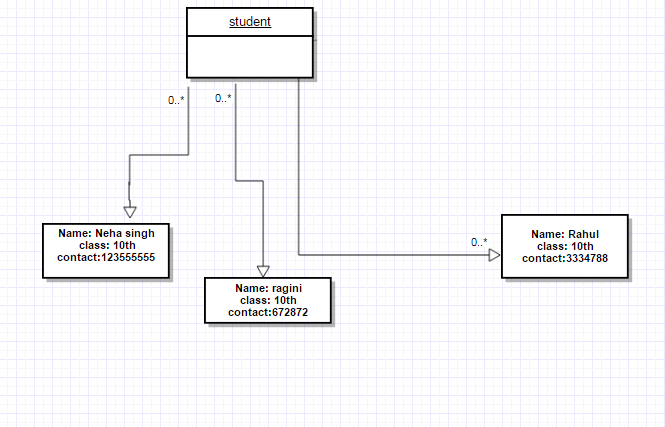##### Package Diagram

Package diagram are termed as subset of class diagram but sometimes developers treat them as a separate technique. The most common use of package diagram is to organize use case diagrams and class diagrams.

Package diagrams can be built either physical or logical relationship. Package diagram allows you to organize model elements into groups, which makes UML diagrams simpler and easy to understand. Packages represents the file folder that can be used on any UML diagrams, they are most common in use case diagrams and class diagrams as they have tendency to grow. Following is an example of package diagram:##### Component diagram

The nature and behavior are different of component diagram. These diagrams are used to model the physical aspects (like executable, files, documents, libraries, etc) of the system. The purpose of component diagram is different from other diagrams in UML. It does not describe the functionality of the system rather describes the components used to make those functionality.

Component diagrams are the static view of a system.  Static view represents the organization of the components at a particular time. A single component diagram is not used to represents the entire system but a collection of diagrams represents the whole system.

These diagrams are used at the time of implementation phase of an application. This diagram is necessary because application cannot be implemented efficiently. Following is an example of component diagram: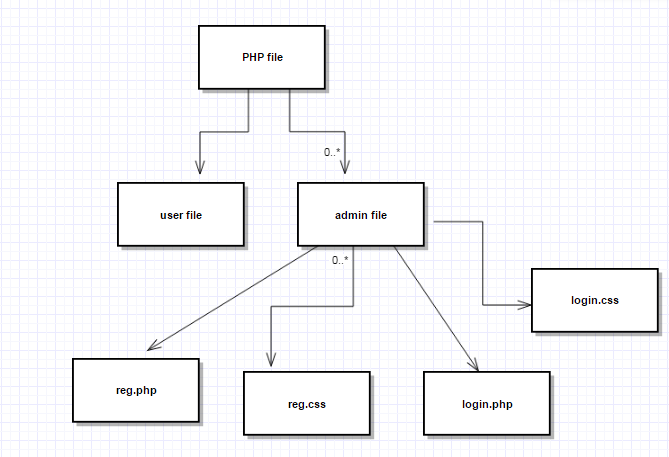##### Composite structural diagram

Composite diagram are used to show the internal structure of a classifier. It also shows the classifier interaction with environments. The term “structure” of a classifier means the composition of interconnected elements, represents the run-time instance that collaborates over communication links to achieve objectives. In other words, composite diagram show the internal part of the program.

Composite structure diagram is a static structure that shows the internal structure of a class and interaction points to other parts of the application. This diagram includes the internal parts, ports through which they interact with each other and outside world. This diagram is a set of interconnected elements that combine at runtime to achieve objectives.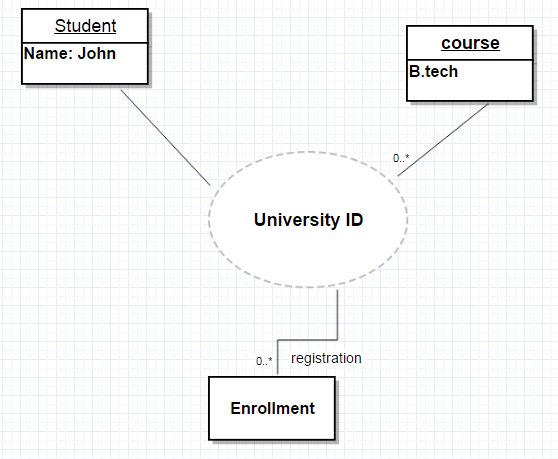##### Deployment diagram

Deployment diagram is the static implementation view of the system. Deployment diagram describes the physical resources of a system like components, nodes and connection. This diagram is used to visualize the topology of the physical component. These diagrams consist of nodes and their relationship. In other words, deployment diagram shows the hardware of your system, where software are deployed.

Deployments diagram and component diagram are closely related. Component diagram describes the components of the system and deployment shows how they are deployed in hardware.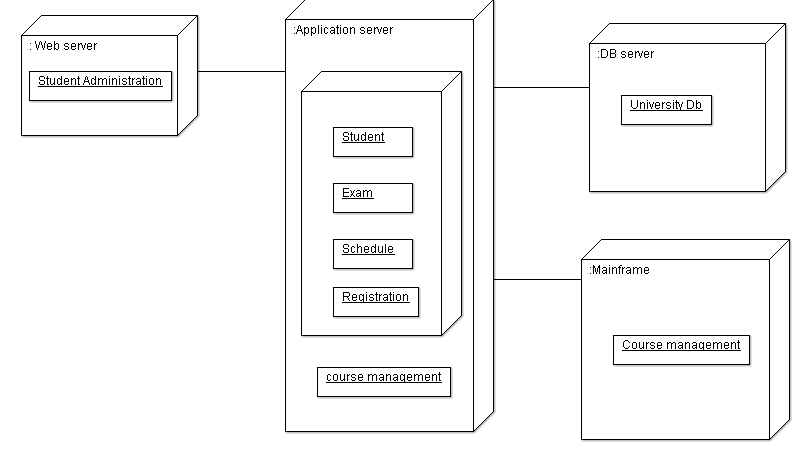##### Activity diagram

Activity diagram is the dynamic view of the system. It is used to show message flow from one activity to another. This flow can be branched or concurrent, sequential. In other words, an activity diagram is a flow chart which represents the flow from one activity to another activity or describe as an operation of the system.

Activity diagram describes the operation of the system. These diagrams are not only used for dynamic visualization but also used to construct executable system using forward and reverse engineering techniques.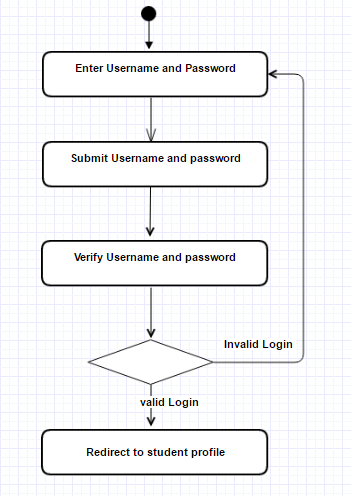##### Sequence diagram

Sequence diagram are also known as event diagram. These diagrams describe the communication between the classes by exchanging messages over time. It is a good way to validate and visualize various runtime scenarios.

These diagrams focus on the message communication between the numbers of lifelines. Sequence diagram describes the communication on the basis of sequence of messages that are exchanged. These diagrams are the most important UML for dynamic modeling, which focuses on the identifying behavior within your system.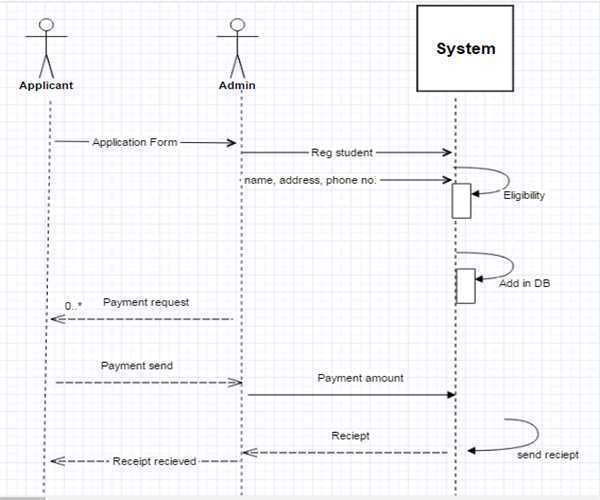##### Use case diagram

Use case diagram describes the dynamic behavior of the object. By dynamic behavior it means the behavior of the system at running or operating.  Use case diagrams, shows the functionality of the system using use cases and actors. Actions, services and functions are the set of use cases. The “actor” is entities or people having defined roles within the system.

Symbols and notation used in Use case diagram

System:  System boundary is drawn using rectangle having use cases. Actor is places outside the rectangle or system.

Use case: use case is drawn in oval shape. Label the oval that represents the system functions.

Actor:-It is the user of the system.

Relationship: It is a relationship between an actor and use case. A relationship can be include and extends. An arrow is labeled with include or extends.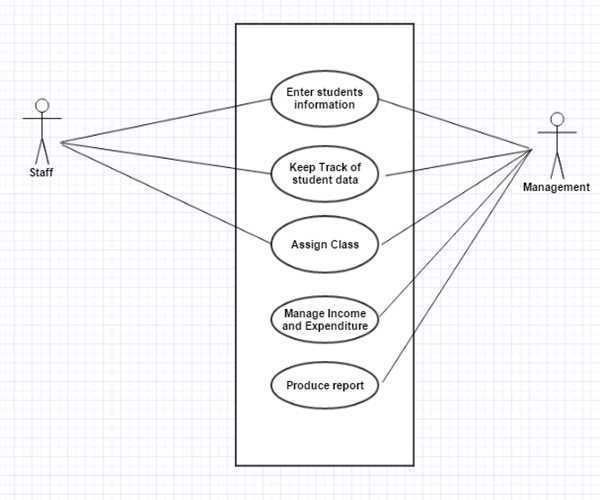##### State chart diagram

State chart diagrams are also known as state machine diagram and state diagram. These diagrams describe the behavior of the system. State diagram are used in reactive objects whose states are triggered by specific events.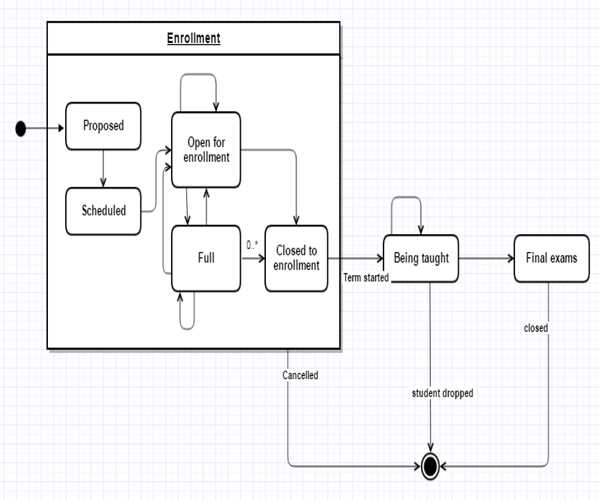##### Communication diagram

Communication diagram shows the interaction between the objects in sequence. These diagrams describe both static view and dynamic view of the system.  Communication diagram, formerly known as collaboration diagrams.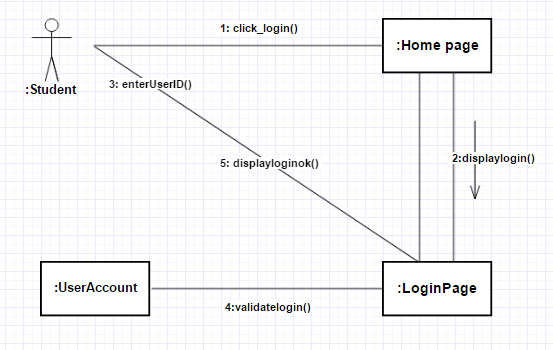##### Interaction overview diagram

Interaction overview diagram are the combination of sequence diagram and activity diagram. These diagram model a sequence of actions and deconstruct more complex interaction into manageable occurrence. The notation used in activity diagram, same notation should be used in interaction diagram.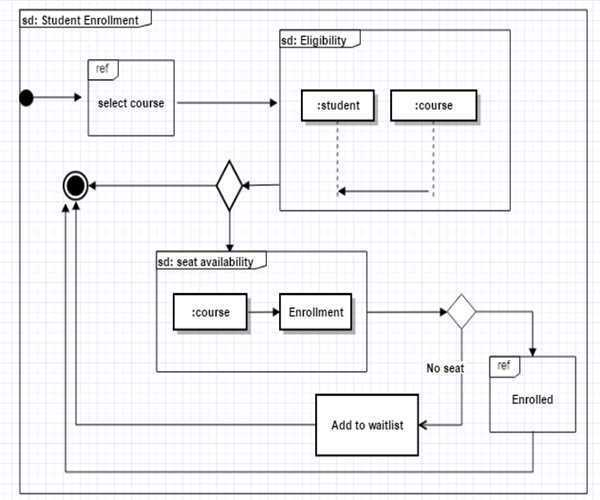##### Timing diagram

A timing diagram is a type of interaction UML diagram that focuses on processes during specific period of time. A timing diagram is an instance of a sequence diagram, except time which is shown from left to right instead of top down.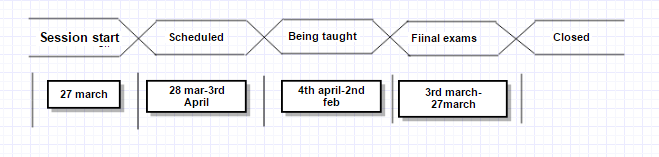Last updated:9/7/2019 12:16:22 AM#### 1 Comments##### Sunil Singh

Hi jonas thanks for sharing good knowladge about unified modeling language